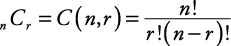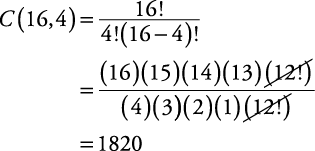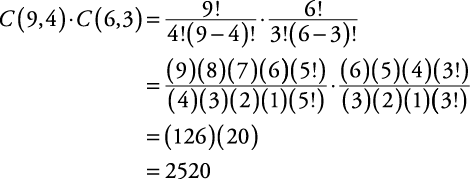## Combinations

When the order in which objects are chosen is not important, as it is in a permutation, the arrangement is called a combination. The combination of n things taken r at a time is written as C(n, r) or nC r . A formula for finding the number of combinations of n objects taken r at a time is given by the following:Note that this is the same as the binomial coefficient formula.

##### Example 1

A class has 16 students. How many groups consisting of four students can be formed?

This is a combinations problem, since the order in which the students in a group are chosen is not important. In this problem, n = 16 and r = 4.There are 1820 different ways to form the groups.

##### Example 2

A committee in Congress consists of nine Republicans and six Democrats. In how many ways can a subcommittee be chosen if it must contain four Republicans and three Democrats?

There are C(9, 4) ways of choosing the four Republicans out of the nine Republicans. There are C(6, 3) ways of choosing the three Democrats out of the six Democrats. By the multiplication principle of events, there are C(9, 4) · C(6, 3) ways of choosing the subcommittee.There are 2520 different possible subcommittees.